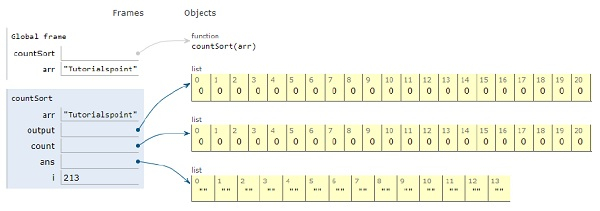# Python Program for Counting Sort

Problem statement− We are given an array, we need to sort the array using the concept of counting sort.

Counting sort is a technique in which we work on keys between a specific range. It involves counting the number of objects which have distinct key & values. Finally, we do arithmetic calculations to obtain the position of each object and display the output.

Now let’s observe the solution in the implementation below −

## Example

Live Demo

def countSort(arr):
# The output character array that will have sorted arr
output = [0 for i in range(256)]
# Create a count array initialized with 0
count = [0 for i in range(256)]
# as strings are immutable
ans = ["" for _ in arr]
# count
for i in arr:
count[ord(i)] += 1
# position of character in the output array
for i in range(256):
count[i] += count[i-1]
# output character array
for i in range(len(arr)):
output[count[ord(arr[i])]-1] = arr[i]
count[ord(arr[i])] -= 1
# array of sorted charcters
for i in range(len(arr)):
ans[i] = output[i]
return ans
# main
arr = "Tutorialspoint"
ans = countSort(arr)
print ("Sorted character array is "+str("".join(ans)))

## Output −

Sorted character array is TaiilnooprsttuAll the variables are declared in the local scope and their references are seen in the figure above.

## Conclusion

In this article, we have learned about how we can make a Python Program for Counting Sort